Array

In the first line below we get a list of Cos[x/4] sampled at
x={1, 2, 3, ... 12}.  You can get the same result using Cos[Range[1/4,3,1/4]], and timing tests show it's faster to do this using Range.  If you are concerned about speed, you should use Range whenever possible.  The #& notation is explained under Function.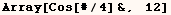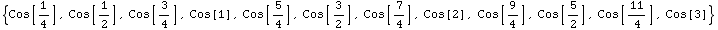The next line gives 12 samples of Cos[x/4] starting at (x=4).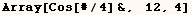Array can take a fourth argument which should be applied to the result instead of list.  The next line finds the minimum of the samples given in the previous line.  Notice the function to be applied must be the fourth argument, so you must provide an starting value as a third argument.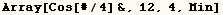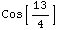The starting place for Array doesn't have to be an integer.  In the next line Array starts at the symbol (t) and samples Cos[_] at 11 points.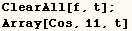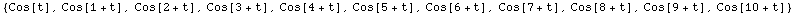In the next line Array is used to make a matrix.  Range can do many things Array can do very efficiently.  However, Array is the method of choice for making matrices such as the following.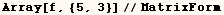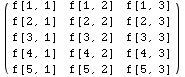In the line above  both arguments of (f) start at one (the default).
Using the next line both arguments of (f) start at 10.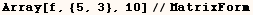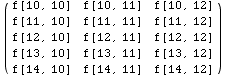In the next line the array starts with 4 in the first argument and 10 in the second argument and counts from there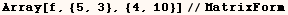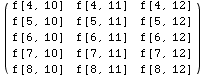Created by Mathematica  (May 16, 2004)

Back to Ted’s Tricks index page